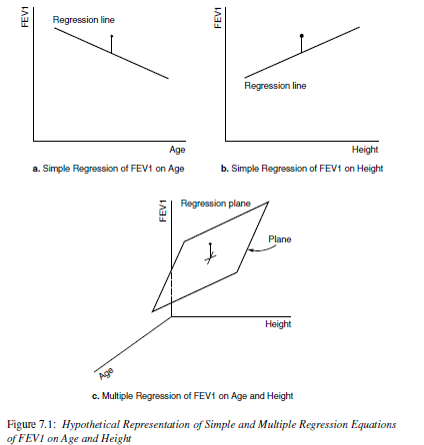# Chapter 9 Multiple Linear Regression

Hopefully by now you have some motivation for why we need to have a robust model that can incorporate information from multiple variables at the same time. Multiple linear regression is our tool to expand our MODEL to better fit the DATA.

• Extends simple linear regression.
• Describes a linear relationship between a single continuous $$Y$$ variable, and several $$X$$ variables.
• Predicts $$Y$$ from $$X_{1}, X_{2}, \ldots , X_{P}$$.
• X’s can be continuous or discrete (categorical)
• X’s can be transformations of other X’s, e.g., $$log(x), x^{2}$$.

Now it’s no longer a 2D regression line, but a $$p$$ dimensional regression plane.This section uses functions from the dotwhisker and gtsummary visualize results from multiple regression models.